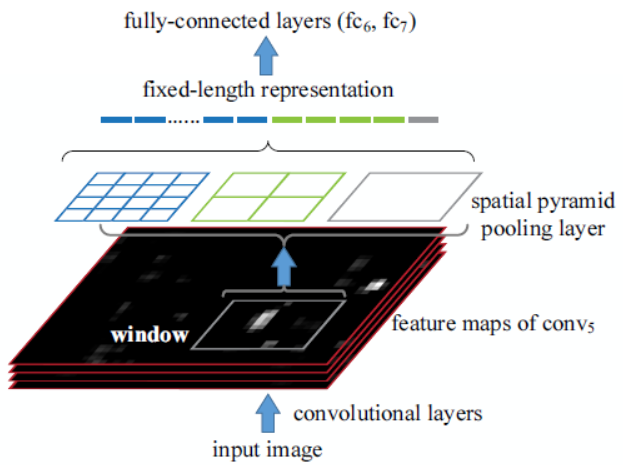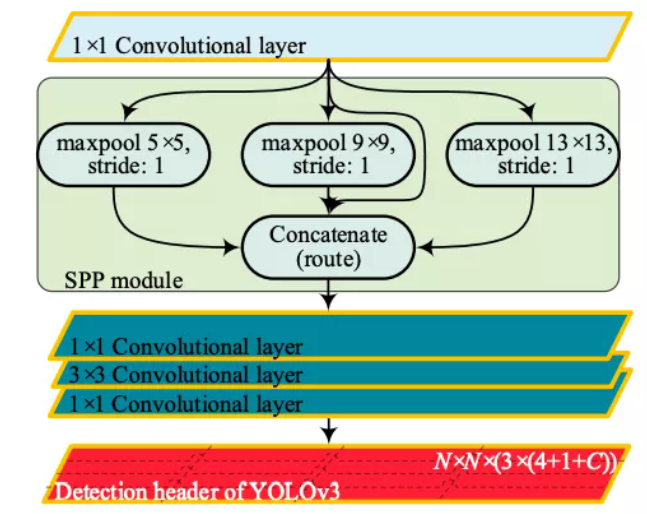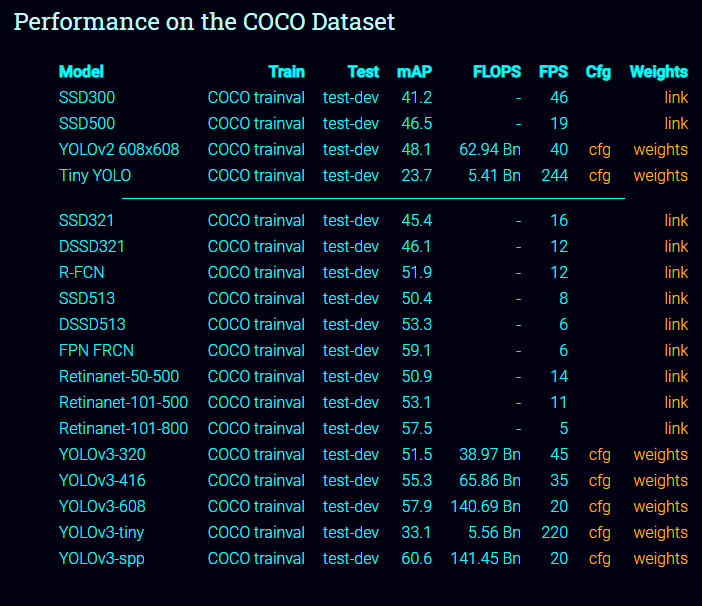# 我们是如何改进YOLOv3进行红外小目标检测的？¶

【GiantPandCV导语】本文将介绍BBuf、小武和笔者一起在过年期间完成的一个目标检测项目，将描述我们模型改进的思路、实验思路、结果汇总和经验性总结。声明：这篇文章经过了三人同意，并且所有创新点也将被公布。此外，由于经验上的不足，可能整个实验思路不够成熟，比不上CV大组的严谨性和完备性，如有问题还烦请指教。

## 1. 红外小目标检测¶trees 581 背景干净，目标明显，数量较多 0.99+0.97
cloudless_sky 1320 背景干净，目标明显，数量多 0.98+0.99
architecture 506 背景变化较大，目标形态变化较大，数量较多 一般 0.92+0.96 focal loss
continuous_cloud_sky 878 背景干净，目标形态变化不大，但个别目标容易会发生和背景中的云混淆 一般 0.93+0.95 focal loss
complex_cloud 561 目标形态基本无变化，但背景对目标的定位影响巨大 较难 0.85+0.89 focal loss
sea 17 背景干净，目标明显，数量极少 一般 0.87+0.88 生成高质量新样本，可以让其转为简单样本（Mixup）
sea_sky 45 背景变化较大，且单张图像中目标个数差异变化大，有密集的难点，且数量少 困难 0.68+0.77 paste策略

## 2. 实验过程¶

### 2.1 修改Anchor¶

#coding=utf-8
import xml.etree.ElementTree as ET
import numpy as np

def iou(box, clusters):
"""
计算一个ground truth边界盒和k个先验框(Anchor)的交并比(IOU)值。
参数box: 元组或者数据，代表ground truth的长宽。
参数clusters: 形如(k,2)的numpy数组，其中k是聚类Anchor框的个数
返回：ground truth和每个Anchor框的交并比。
"""
x = np.minimum(clusters[:, 0], box)
y = np.minimum(clusters[:, 1], box)
if np.count_nonzero(x == 0) > 0 or np.count_nonzero(y == 0) > 0:
raise ValueError("Box has no area")
intersection = x * y
box_area = box * box
cluster_area = clusters[:, 0] * clusters[:, 1]
iou_ = intersection / (box_area + cluster_area - intersection)
return iou_

def avg_iou(boxes, clusters):
"""
计算一个ground truth和k个Anchor的交并比的均值。
"""
return np.mean([np.max(iou(boxes[i], clusters)) for i in range(boxes.shape)])

def kmeans(boxes, k, dist=np.median):
"""
利用IOU值进行K-means聚类
参数boxes: 形状为(r, 2)的ground truth框，其中r是ground truth的个数
参数k: Anchor的个数
参数dist: 距离函数
返回值：形状为(k, 2)的k个Anchor框
"""
# 即是上面提到的r
rows = boxes.shape
# 距离数组，计算每个ground truth和k个Anchor的距离
distances = np.empty((rows, k))
# 上一次每个ground truth"距离"最近的Anchor索引
last_clusters = np.zeros((rows,))
# 设置随机数种子
np.random.seed()

# 初始化聚类中心，k个簇，从r个ground truth随机选k个
clusters = boxes[np.random.choice(rows, k, replace=False)]
# 开始聚类
while True:
# 计算每个ground truth和k个Anchor的距离，用1-IOU(box,anchor)来计算
for row in range(rows):
distances[row] = 1 - iou(boxes[row], clusters)
# 对每个ground truth，选取距离最小的那个Anchor，并存下索引
nearest_clusters = np.argmin(distances, axis=1)
# 如果当前每个ground truth"距离"最近的Anchor索引和上一次一样，聚类结束
if (last_clusters == nearest_clusters).all():
break
# 更新簇中心为簇里面所有的ground truth框的均值
for cluster in range(k):
clusters[cluster] = dist(boxes[nearest_clusters == cluster], axis=0)
# 更新每个ground truth"距离"最近的Anchor索引
last_clusters = nearest_clusters

return clusters

# 加载自己的数据集，只需要所有labelimg标注出来的xml文件即可
dataset = []
for xml_file in glob.glob("{}/*xml".format(path)):
tree = ET.parse(xml_file)
# 图片高度
height = int(tree.findtext("./size/height"))
# 图片宽度
width = int(tree.findtext("./size/width"))

for obj in tree.iter("object"):
# 偏移量
xmin = int(obj.findtext("bndbox/xmin")) / width
ymin = int(obj.findtext("bndbox/ymin")) / height
xmax = int(obj.findtext("bndbox/xmax")) / width
ymax = int(obj.findtext("bndbox/ymax")) / height
xmin = np.float64(xmin)
ymin = np.float64(ymin)
xmax = np.float64(xmax)
ymax = np.float64(ymax)
if xmax == xmin or ymax == ymin:
print(xml_file)
# 将Anchor的长宽放入dateset，运行kmeans获得Anchor
dataset.append([xmax - xmin, ymax - ymin])
return np.array(dataset)

if __name__ == '__main__':

ANNOTATIONS_PATH = "F:\Annotations" #xml文件所在文件夹
CLUSTERS = 9 #聚类数量，anchor数量
INPUTDIM = 416 #输入网络大小

out = kmeans(data, k=CLUSTERS)
print('Boxes:')
print(np.array(out)*INPUTDIM)
print("Accuracy: {:.2f}%".format(avg_iou(data, out) * 100))
final_anchors = np.around(out[:, 0] / out[:, 1], decimals=2).tolist()
print("Before Sort Ratios:\n {}".format(final_anchors))
print("After Sort Ratios:\n {}".format(sorted(final_anchors)))


def kmean_anchors(path='./2007_train.txt', n=5, img_size=(416, 416)):
# from utils.utils import *; _ = kmean_anchors()
# Produces a list of target kmeans suitable for use in *.cfg files
thr = 0.20  # IoU threshold

def print_results(thr, wh, k):
k = k[np.argsort(k.prod(1))]  # sort small to large
iou = wh_iou(torch.Tensor(wh), torch.Tensor(k))
max_iou, min_iou = iou.max(1), iou.min(1)
bpr, aat = (max_iou > thr).float().mean(), (
iou > thr).float().mean() * n  # best possible recall, anch > thr
print('%.2f iou_thr: %.3f best possible recall, %.2f anchors > thr' %
(thr, bpr, aat))
print(
'kmeans anchors (n=%g, img_size=%s, IoU=%.3f/%.3f/%.3f-min/mean/best): '
% (n, img_size, min_iou.mean(), iou.mean(), max_iou.mean()),
end='')
for i, x in enumerate(k):
print('%i,%i' % (round(x), round(x)),
end=',  ' if i < len(k) - 1 else '\n')  # use in *.cfg
return k

def fitness(thr, wh, k):  # mutation fitness
iou = wh_iou(wh, torch.Tensor(k)).max(1)  # max iou
bpr = (iou > thr).float().mean()  # best possible recall
return iou.mean() * bpr  # product

# Get label wh
wh = []
augment=True,
rect=True,
cache_labels=True)
nr = 1 if img_size == img_size else 10  # number augmentation repetitions
for s, l in zip(dataset.shapes, dataset.labels):
wh.append(l[:, 3:5] *
(s / s.max()))  # image normalized to letterbox normalized wh
wh = np.concatenate(wh, 0).repeat(nr, axis=0)  # augment 10x
wh *= np.random.uniform(img_size, img_size,
size=(wh.shape,
1))  # normalized to pixels (multi-scale)

# Darknet yolov3.cfg anchors
use_darknet = False
if use_darknet:
k = np.array([[10, 13], [16, 30], [33, 23], [30, 61], [62, 45],
[59, 119], [116, 90], [156, 198], [373, 326]])
else:
# Kmeans calculation
from scipy.cluster.vq import kmeans
print('Running kmeans for %g anchors on %g points...' % (n, len(wh)))
s = wh.std(0)  # sigmas for whitening
k, dist = kmeans(wh / s, n, iter=30)  # points, mean distance
k *= s
k = print_results(thr, wh, k)
# Evolve
wh = torch.Tensor(wh)
f, ng = fitness(thr, wh, k), 2000  # fitness, generations
for _ in tqdm(range(ng), desc='Evolving anchors'):
kg = (
k.copy() *
(1 + np.random.random() * np.random.randn(*k.shape) * 0.30)).clip(
min=2.0)
fg = fitness(thr, wh, kg)
if fg > f:
f, k = fg, kg.copy()
print_results(thr, wh, k)
k = print_results(thr, wh, k)

return k


Anchor这方面设置了三个不同的数量进行聚类：

3 anchor:

13, 18, 16, 22, 19, 25


6 anchor:

12,17, 14,17, 15,19, 15,21, 13,20, 19,24


9 anchor:

10,16, 12,17, 13,20, 13,22, 15,18, 15,20, 15,23, 18,23, 21,26


### 2.2 构建Baseline¶

Epoch Model P R mAP@0.5 F1 dataset
baseline yolov3-tiny原版 0.982 0.939 0.932 0.96 valid
baseline yolov3-tiny原版 0.96 0.873 0.869 0.914 test
6a yolov3-tiny-6a 0.973 0.98 0.984 0.977 valid
6a yolov3-tiny-6a 0.936 0.925 0.915 0.931 test

### 2.3 数据集部分改进¶

Epoch Model P R mAP@0.5 F1 dataset
baseline(os) yolov3-tiny原版 0.985 0.971 0.973 0.978 valid
baseline(os) yolov3-tiny原版 0.936 0.871 0.86 0.902 test
baseline yolov3-tiny原版 0.982 0.939 0.932 0.96 valid
baseline yolov3-tiny原版 0.96 0.873 0.869 0.914 test

:( 可惜实验结果不支持想法，一起分析一下。ps:os代表over sample

data num model P R mAP F1
trees 506 yolov3-tiny-6a 0.924 0.996 0.981 0.959
sea_sky 495 yolov3-tiny-6a 0.927 0.978 0.771 0.85
sea 510 yolov3-tiny-6a 0.923 0.935 0.893 0.929
continuous_cloud_sky 878 yolov3-tiny-6a 0.957 0.95 0.933 0.953
complex_cloud 561 yolov3-tiny-6a 0.943 0.833 0.831 0.885
cloudless_sky 1320 yolov3-tiny-6a 0.993 0.981 0.984 0.987
architecture 506 yolov3-tiny-6a 0.959 0.952 0.941 0.955### 2.4 修改Backbone¶

• 干脆不加载，从头训练，简单问题（比如红外小目标）从头收敛效果也不次于有预训练权重的。

• 能力比较强的，可以改一下模型加载部分代码，跳过你新加入的模块，这样也能加载（笔者没试过，别找我）。

1. 注意力模块

2. 即插即用模块

3. 修改FPN

FPN这方面花了老久时间，参考了好多版本才搞出了一个dt-6a-bifpn(dt代表dim target红外目标；6a代表6个anchor)，令人失望的是，这个BiFPN效果并不好，测试集上效果更差了。可能是因为实现的cfg有问题，欢迎反馈。

https://lutzroeder.github.io/netron/


import os
import shutil
cfg_path = "./cfg/yolov3-dwconv-cbam.cfg"
save_path = "./cfg/preprocess_cfg/"
new_save_name = os.path.join(save_path,os.path.basename(cfg_path))

f = open(cfg_path, 'r')

# 去除以#开头的，属于注释部分的内容
# lines = [x for x in lines if x and not x.startswith('#')]
# lines = [x.rstrip().lstrip() for x in lines]

lines_nums = []
layers_nums = []

layer_cnt = -1

for num, line in enumerate(lines):
if line.startswith('['):
layer_cnt += 1
layers_nums.append(layer_cnt)
lines_nums.append(num+layer_cnt)
print(line)
# s = s.join("")
# s = s.join(line)
for i,num in enumerate(layers_nums):
print(lines_nums[i], num)
lines.insert(lines_nums[i]-1, '# layer-%d\n' % (num-1))
fo = open(new_save_name, 'w')
fo.write(''.join(lines))
fo.close()
f.close()


4. 修改激活函数

YOLO默认使用的激活函数是leaky relu,激活函数方面使用了mish。效果并没有提升，所以无疾而终了。

5. 用成熟的网络替换backbone

6. SPP系列

SPP是在SPPNet中提出的，SPPNet提出比较早，在RCNN之后提出的，用于解决重复卷积计算和固定输出的两个问题，具体方法如下图所示：### SPP ###
[maxpool]
stride=1
size=5

[route]
layers=-2

[maxpool]
stride=1
size=9

[route]
layers=-4

[maxpool]
stride=1
size=13

[route]
layers=-1,-3,-5,-6

### End SPP ###1. 从感受野角度来讲，之前计算感受野的时候可以明显发现，maxpool的操作对感受野的影响非常大，其中主要取决于kernel size大小。在SPP中，使用了kernel size非常大的maxpool会极大提高模型的感受野，笔者没有详细计算过darknet53这个backbone的感受野，在COCO上有效很可能是因为backbone的感受野还不够大。
2. 第二个角度是从Attention的角度考虑，这一点启发自CSDN@小楞，他在文章中这样讲：

Attention机制很多都是为了解决远距离依赖问题，通过使用kernel size接近特征图的size可以以比较小的计算代价解决这个问题。另外就是如果使用了SPP模块，就没有必要在SPP后继续使用其他空间注意力模块比如SK block，因为他们作用相似，可能会有一定冗余。

SPP是有效的，其中size的设置应该接近这一层的feature map的大小

SPP系列实验

Epoch Model P R mAP F1 dataset
baseline dt-6a-spp 0.99 0.983 0.984 0.987 valid
baseline dt-6a-spp 0.955 0.948 0.929 0.951 test

### 2.5 修改Loss¶

loss方面尝试了focal loss，但是经过调整alpha和beta两个参数，不管用默认的还是自己慢慢调参，网络都无法收敛，所以当时给作者提了一个issue: https://github.com/ultralytics/yolov3/issues/811

glenn-jocher说效果不好就别用:(BBuf也研究了好长时间，发现focal loss在Darknet中可以用，但是效果也一般般。最终focal loss也是无疾而终。此外还试着调整了ignore thresh，来配合focal loss，实验结果如下（在AB版Darknet下完成实验）：

state model P R mAP F1 data
ignore=0.7 dt-6a-spp-fl 0.97 0.97 0.9755 0.97 valid
ignore=0.7 dt-6a-spp-fl 0.96 0.93 0.9294 0.94 test
ignore=0.3 dt-6a-spp-fl 0.95 0.99 0.9874 0.97 valid
ignore=0.3 dt-6a-spp-fl 0.89 0.92 0.9103 0.90 test

## 3. 经验性总结¶

• SPP层是有效的，Size设置接近feature map的时候效果更好。
• YOLOv3、YOLOv3-SPP、YOLOv3-tiny三者在检测同一个物体的情况下，YOLOv3-tiny给的该物体的置信度相比其他两个模型低。（其实也可以形象化理解，YOLOv3-tiny的脑容量比较小，所以唯唯诺诺不敢确定）
• 多尺度训练问题，这个文中没提。多尺度训练对于尺度分布比较广泛的问题效果明显，比如VOC这类数据集。但是对于尺度单一的数据集反而有反作用，比如红外小目标数据集目标尺度比较统一，都很小。
• Anchor对模型影响比较大，Anchor先验不合理会导致更多的失配，从而降低Recall。
• PyTorch中的Upsample层是不可复现的。
• 有卡可以尝试一下超参数进化方法。

PS: 以上内容不保证结论完全正确，只是经验性总结，欢迎入群讨论交流。

## 5. 资源列表¶

Focal Loss Issue: https://github.com/ultralytics/yolov3/issues/811

pprp Github: https://github.com/pprp

BBuf Github:https://github.com/BBuf